## Convert Kilograms to Pounds or Vice Versa in Excel Worksheets

by Avantix Learning Team | Updated October 14, 2022

Applies to: Microsoft® Excel® 2010, 2013, 2016, 2019, 2021 and 365 (Windows)

You can convert kg to lbs (kilograms to pounds) or pounds to kilograms using formulas with operators or functions in Excel.

Recommended article: How to Hide Formulas from Clients, Colleagues and Other Users

Do you want to learn more about Excel? Check out our virtual classroom or in-person classroom Excel courses >

The following are the conversion amounts:

1 pound (lb) = 0.453592 kilograms (kg)

For an approximate result, divide the value or cell value by 2.205.

1 kilogram (kg) = 2.20462 pounds (lb)

For an approximate result, multiply the value or cell value by 2.205.

### Convert kg to lbs (kilograms to pounds) by multiplying

To convert from kg to lbs (kilograms to pounds) by multiplying:

1. Click in a cell where you want to insert the converted amount.
2. Type =cell to convert*2.205 (for example =A2*2.205)
3. Press Enter.

In the following example, the kg to lbs (kilograms to pounds) conversion formula is entered in column B using the multiplication operator: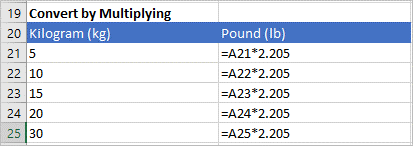### Convert lbs to kg (pounds to kilograms) by dividing

To convert from lbs to kg (pounds to kilograms) by dividing:

1. Click in a cell where you want to insert the converted amount.
2. Type =cell to convert/2.205 (for example =A2/2.205)
3. Press Enter.

In the following example, the lbs to kg (pounds to kilograms) conversion formula is entered in column B using the division operator: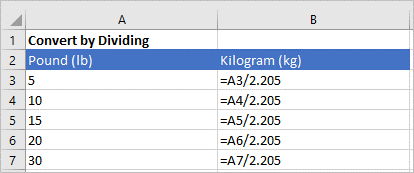### Use the CONVERT function

You can also use the CONVERT function to convert measurements.

The CONVERT function uses the following syntax:

=CONVERT(cell to convert, units to convert from, units to convert to).

The units must be entered in quotation marks or double quotes (such as "kg").

### Convert kg to lbs (kilograms to pounds) using the CONVERT function

To convert kg to lbs (kilograms to pounds) using the CONVERT function:

1. Click in a cell where you want to insert the converted amount.
2. Type =CONVERT(cell to convert,"kg","lbm"). For example, type =CONVERT(A2,"kg","lbm").
3. Press Enter.

In the following example, the kg to lbs (kilograms to pounds) conversion formula is entered in column B using the CONVERT function: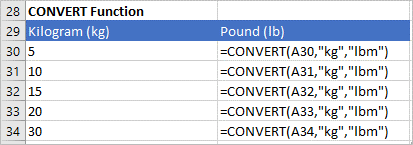### Convert lbs to kg (pounds to kilograms) using the CONVERT function

To convert lbs to kg (pounds to kilograms) using the CONVERT function:

1. Click in a cell where you want to insert the converted amount.
2. Type =CONVERT(cell to convert,"lbm","kg"). For example, type =CONVERT(A2,"lbm","kg").
3. Press Enter.

In the following example, the lbs to kg (pounds to kilograms) conversion formula is entered in column B using the CONVERT function: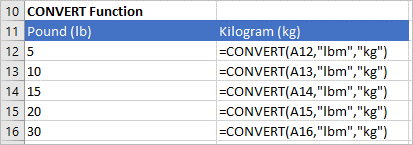There are also other units you can use with the CONVERT function.

This article was first posted on August 14, 2021 and has been updated for clarity and content.

#### More resources

How to Use Flash Fill in Excel (4 Ways with Shortcuts)

3 Excel Strikethrough Shortcuts to Cross Out Text or Values in Cells

How to Highlight Errors, Blanks and Duplicates in Excel Worksheets

How to Replace Blank Cells with a Value from the Cell Above in Excel

#### Related courses

Microsoft Excel: Data Analysis with Functions, Dashboards and What-If Analysis Tools

Microsoft Excel: Introduction to Power Query to Get and Transform Data

Microsoft Excel: New and Essential Features and Functions in Excel 365

Microsoft Excel: Introduction to Visual Basic for Applications (VBA)

VIEW MORE COURSES >

Our instructor-led courses are delivered in virtual classroom format or at our downtown Toronto location at 18 King Street East, Suite 1400, Toronto, Ontario, Canada (some in-person classroom courses may also be delivered at an alternate downtown Toronto location). Contact us at info@avantixlearning.ca if you'd like to arrange custom instructor-led virtual classroom or onsite training on a date that's convenient for you.

## How to Combine First and Last Name in Excel (5 Ways)

You can combine first and last name in Excel in several ways – using the CONCATENATE operator, the CONCATENATE function, the CONCAT function, the TEXTJOIN function or Flash Fill. These functions are often used to combine text in cells but you can also combine text with spaces, commas, dashes or another character. It's common to combine first and last names that appear in two columns into one new column. Some functions are only available in newer versions of Excel but the CONCATENATE operator and function are available in all versions.

## How to Insert Multiple Columns in Excel (4 Fast Ways with Shortcuts)

You can quickly insert multiple columns in Excel using a drop-down menu, keyboard shortcuts or the Home tab in the Ribbon. The key to inserting multiple columns at once, rather than one by one, is to select the number of columns you want to insert first and then insert columns. Excel will insert the same number of columns you selected.

## Excel XLOOKUP Function (Overview, Syntax + Formula Examples)

The XLOOKUP function is a replacement for Excel's traditional VLOOKUP function (as well as HLOOKUP and INDEX / MATCH functions). It has a new set of arguments and is available in Excel 2021 and 365. It allows you to look up a value from an array in a range or table and return one or more results. One of the primary benefits of XLOOKUP is that it can look up from columns to the left in a data set and return a range.

Microsoft, the Microsoft logo, Microsoft Office and related Microsoft applications and logos are registered trademarks of Microsoft Corporation in Canada, US and other countries. All other trademarks are the property of the registered owners.

Avantix Learning |18 King Street East, Suite 1400, Toronto, Ontario, Canada M5C 1C4 | Contact us at info@avantixlearning.ca

Summary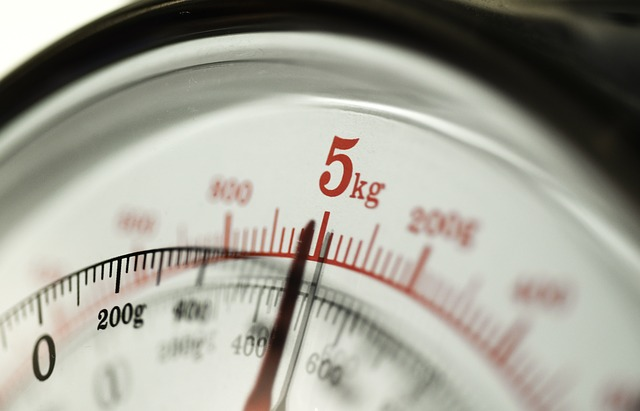Article Name
How to Convert Kg to Lbs (Kilograms to Pounds) in Excel or Lbs to Kg
Description
You can convert kg to lbs (kilograms to pounds) or pounds to kilograms in Excel using formulas with operators or functions.
Author
Publisher Name
Avantix Learning